5740

Mathematics Integration Level: Misc Level

Find the (absolute) maximum and the (absolute) minimum values of the following function in the indicated intervals. Also find the points of (absolute) maxima and minima

f(x) = (x-1)2 + 3 in [-3,1]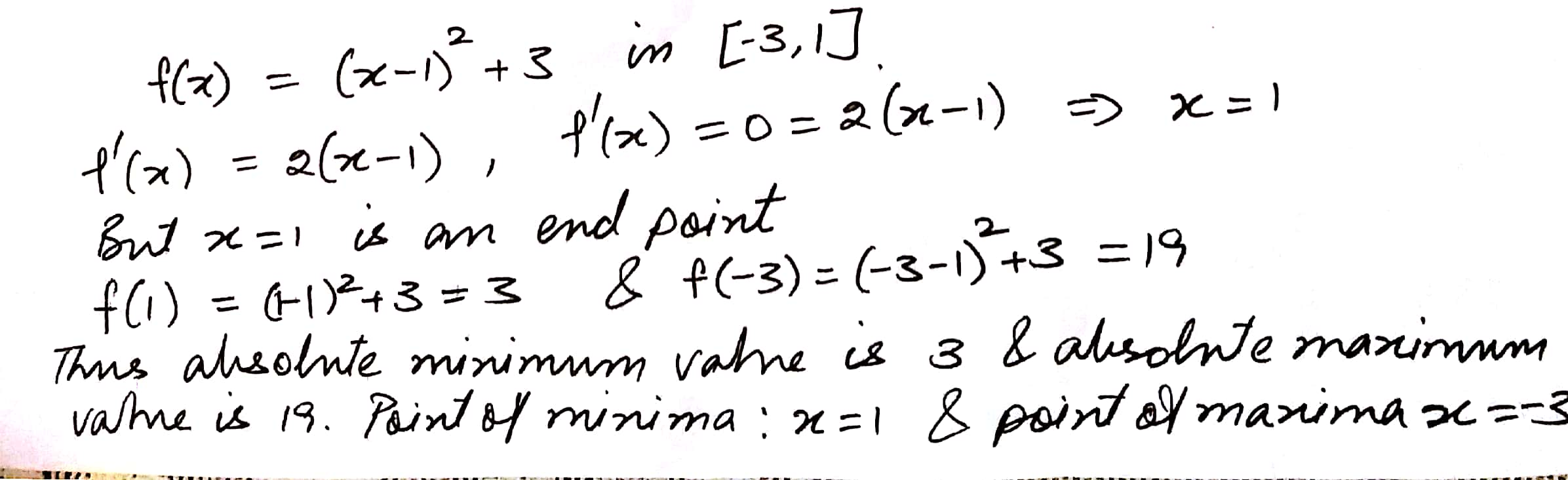5739

Mathematics Integration Level: Misc Level

Find the maximum and the minimum values (if any) of the following function

f(x) = Sin 2x + 5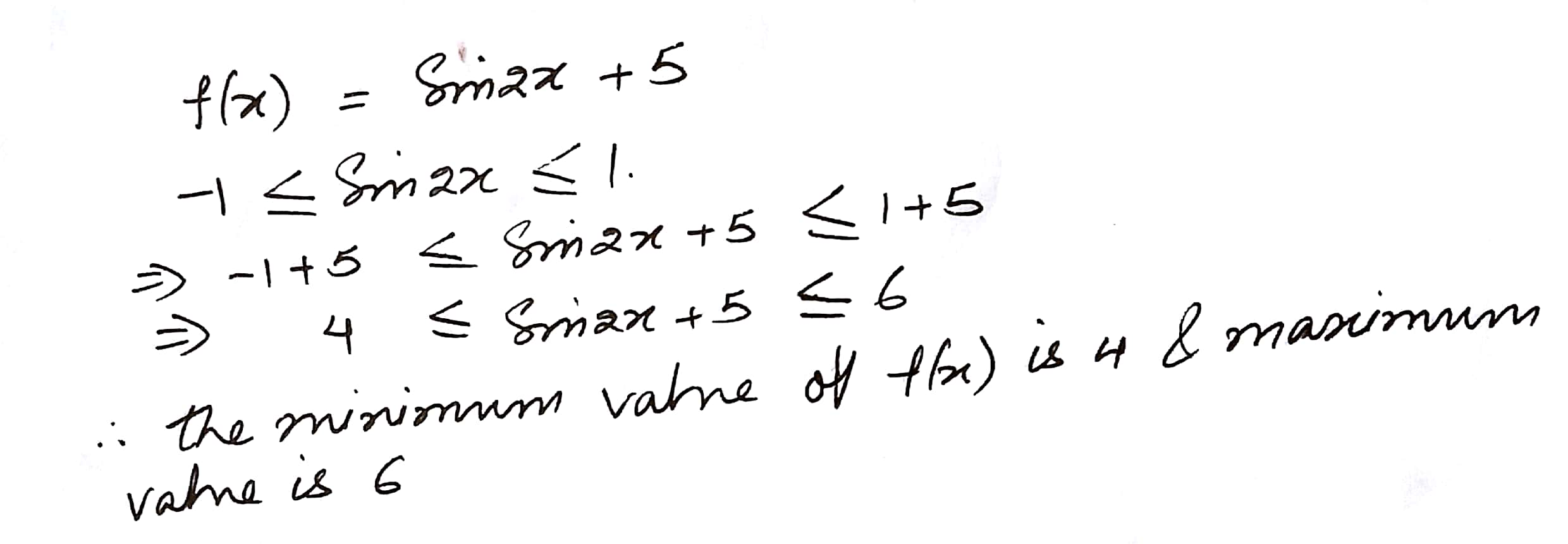5738

Mathematics Integration Level: Misc Level

Find the maximum and the minimum values (if any) of the following function

f(x) = |x + 2| - 1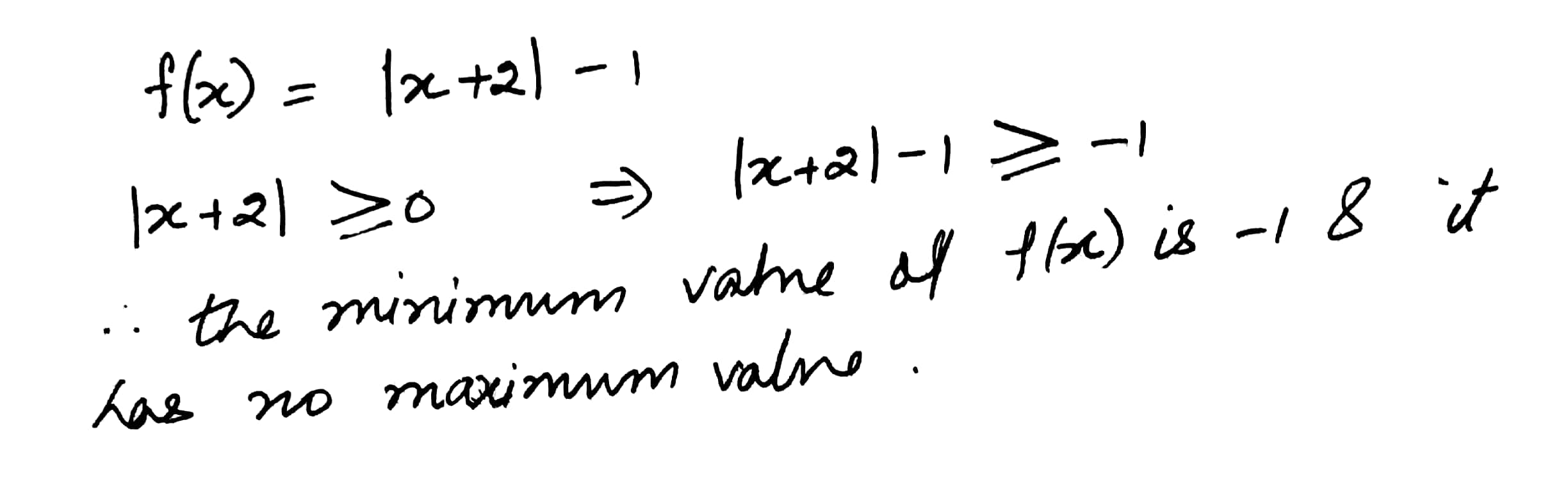5737

Mathematics Integration Level: Misc Level

Find the maximum and the minimum values (if any) of the following function

f(x) = - (x-1)2 + 10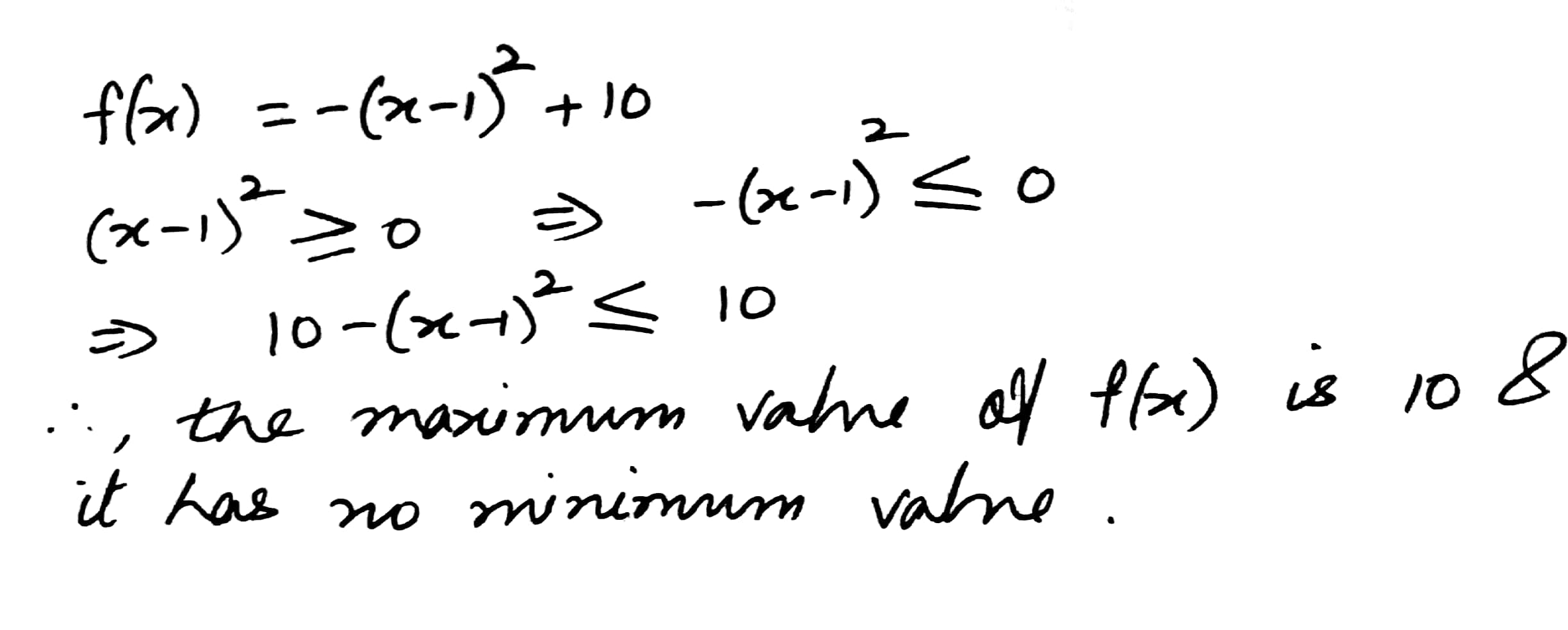5730

Mathematics Integration Level: Misc Level

Find the interval in which f(x) = x2e-x is increasing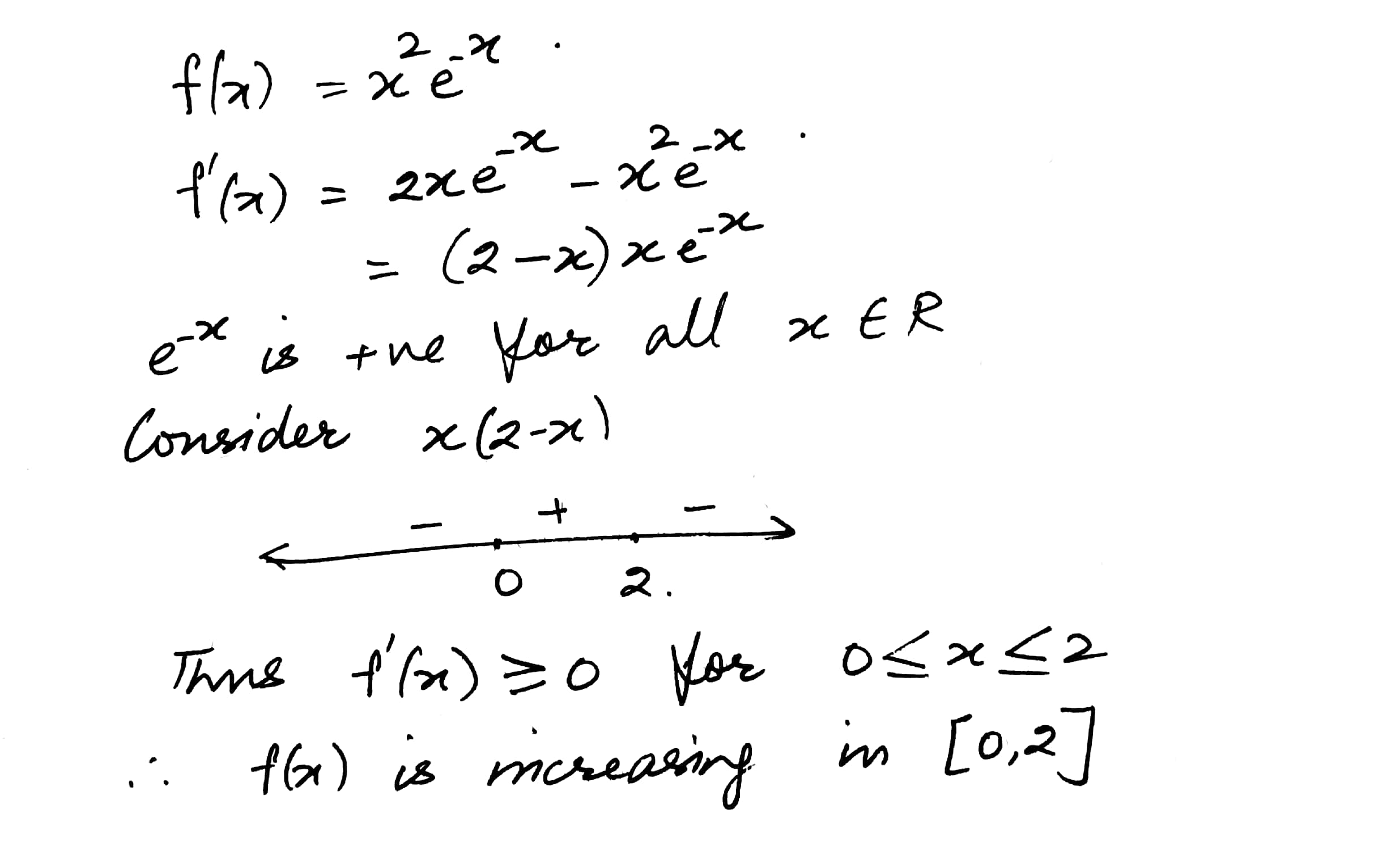5729

Mathematics Integration Level: Misc Level

Find the interval in which the following function is strictly increasing or strictly decreasing

f(x) = x4 - 4x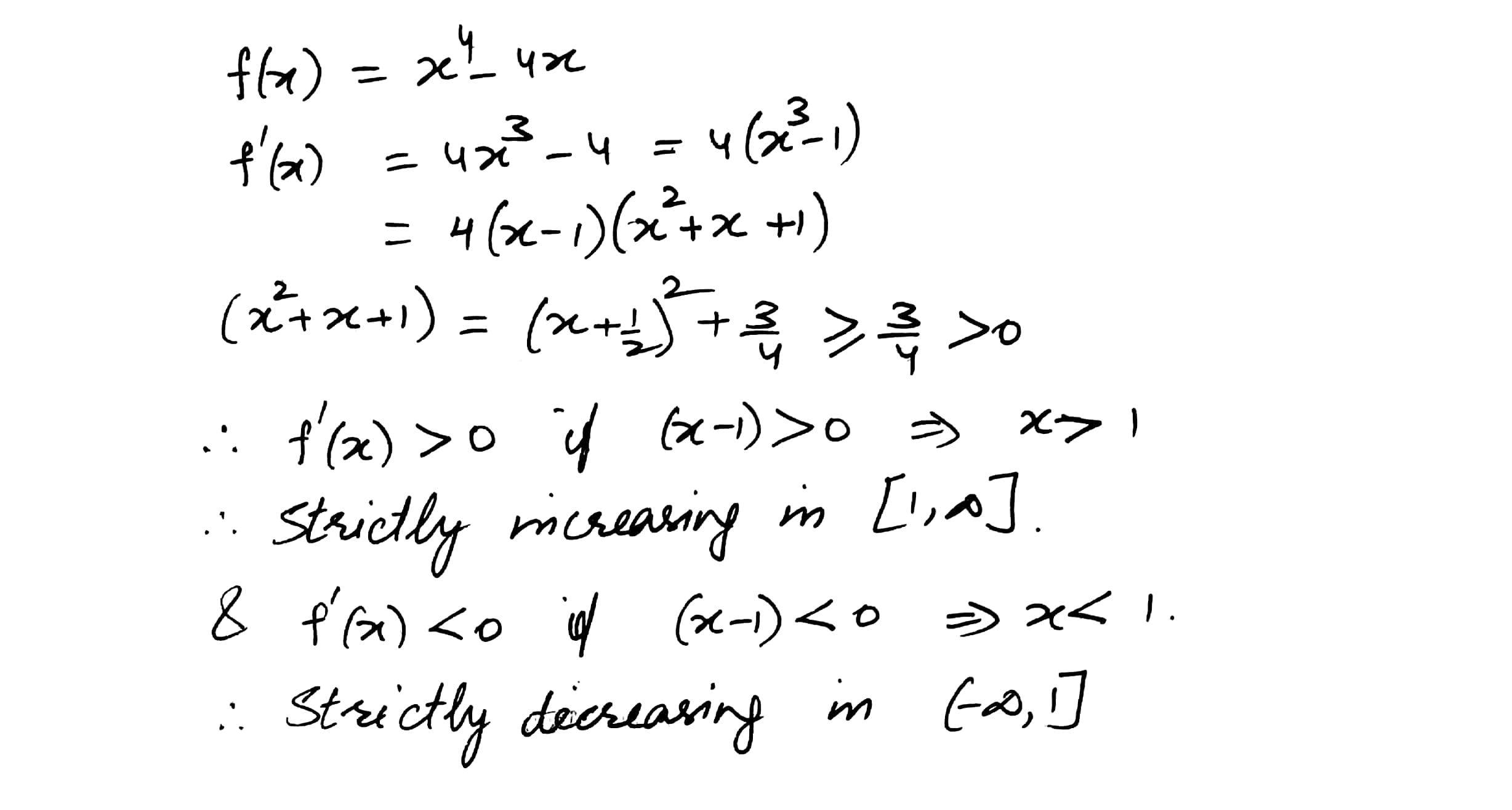5728

Mathematics Integration Level: Misc Level

Find the interval in which the following function is strictly increasing or strictly decreasing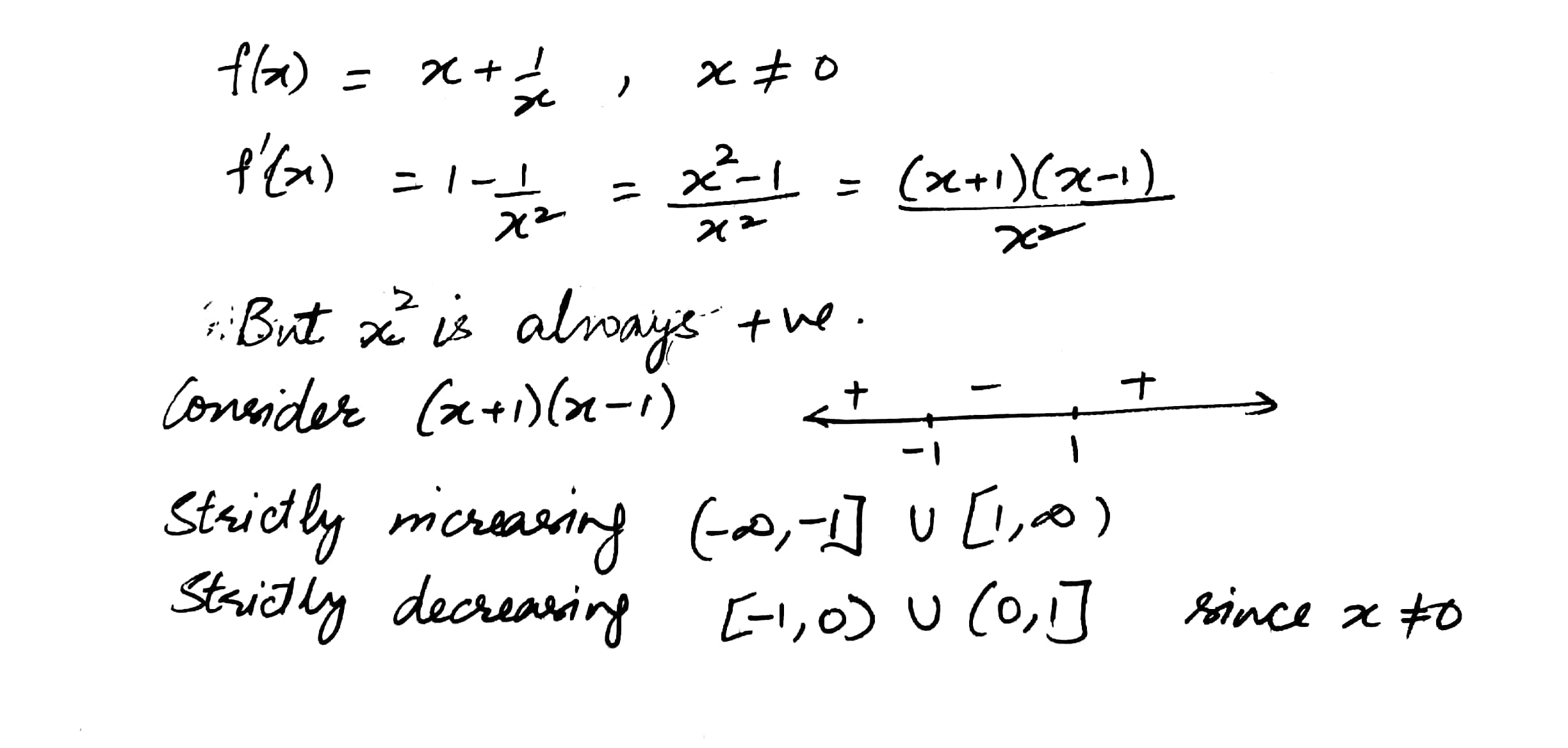5727

Mathematics Integration Level: Misc Level

Find the interval in which the following function is strictly increasing or strictly decreasing

f(x) = 2x3 - 3x2 - 36x + 7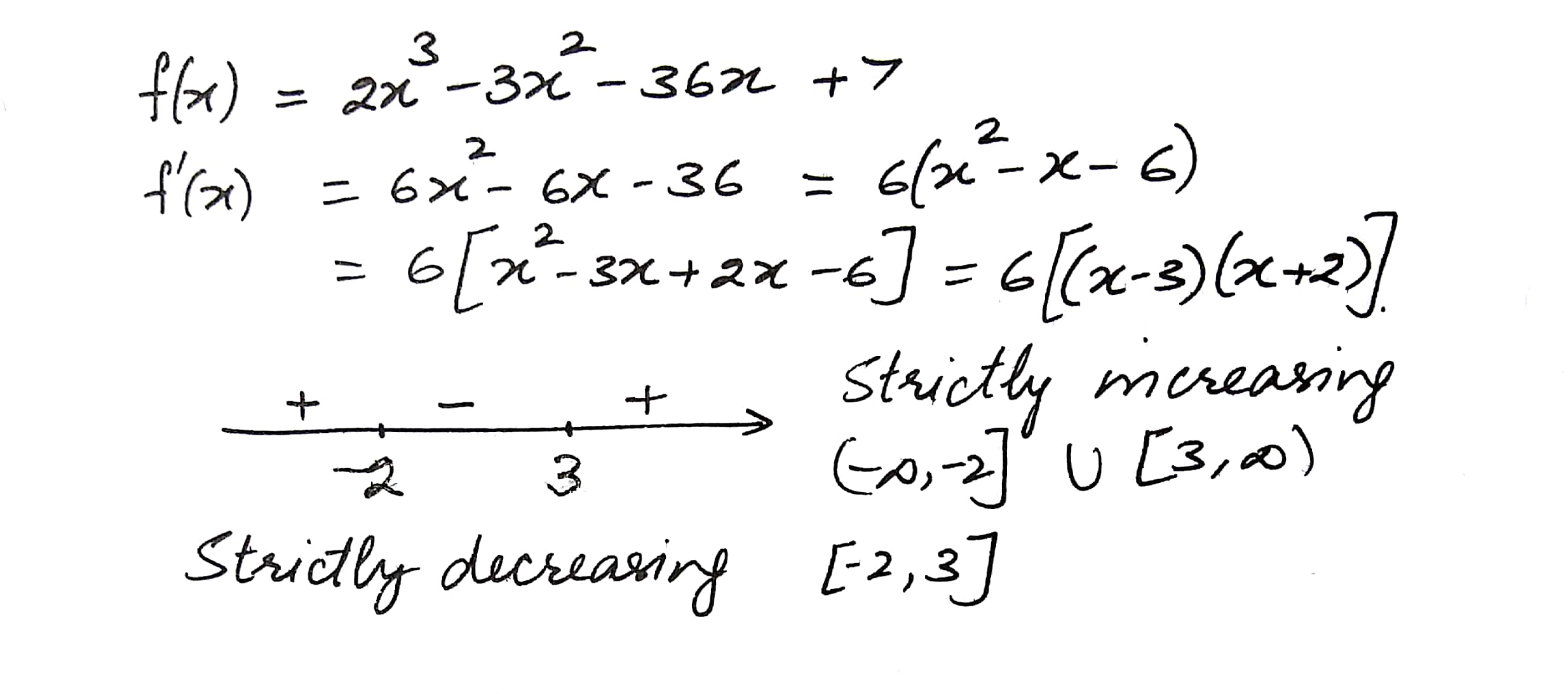5726

Mathematics Integration Level: Misc Level

Find the interval in which the following function is strictly increasing or strictly decreasing

f(x) = x2 - 4x + 6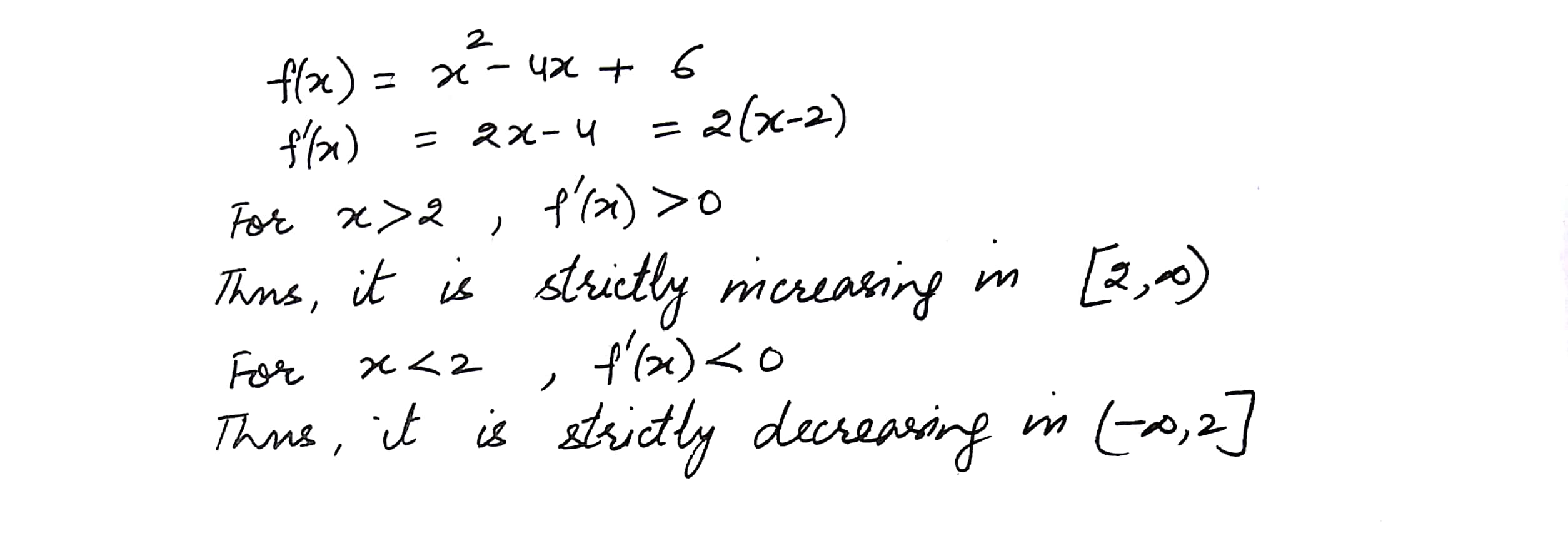5725

Mathematics Integration Level: Misc Level

Prove that the function f given by is increasing in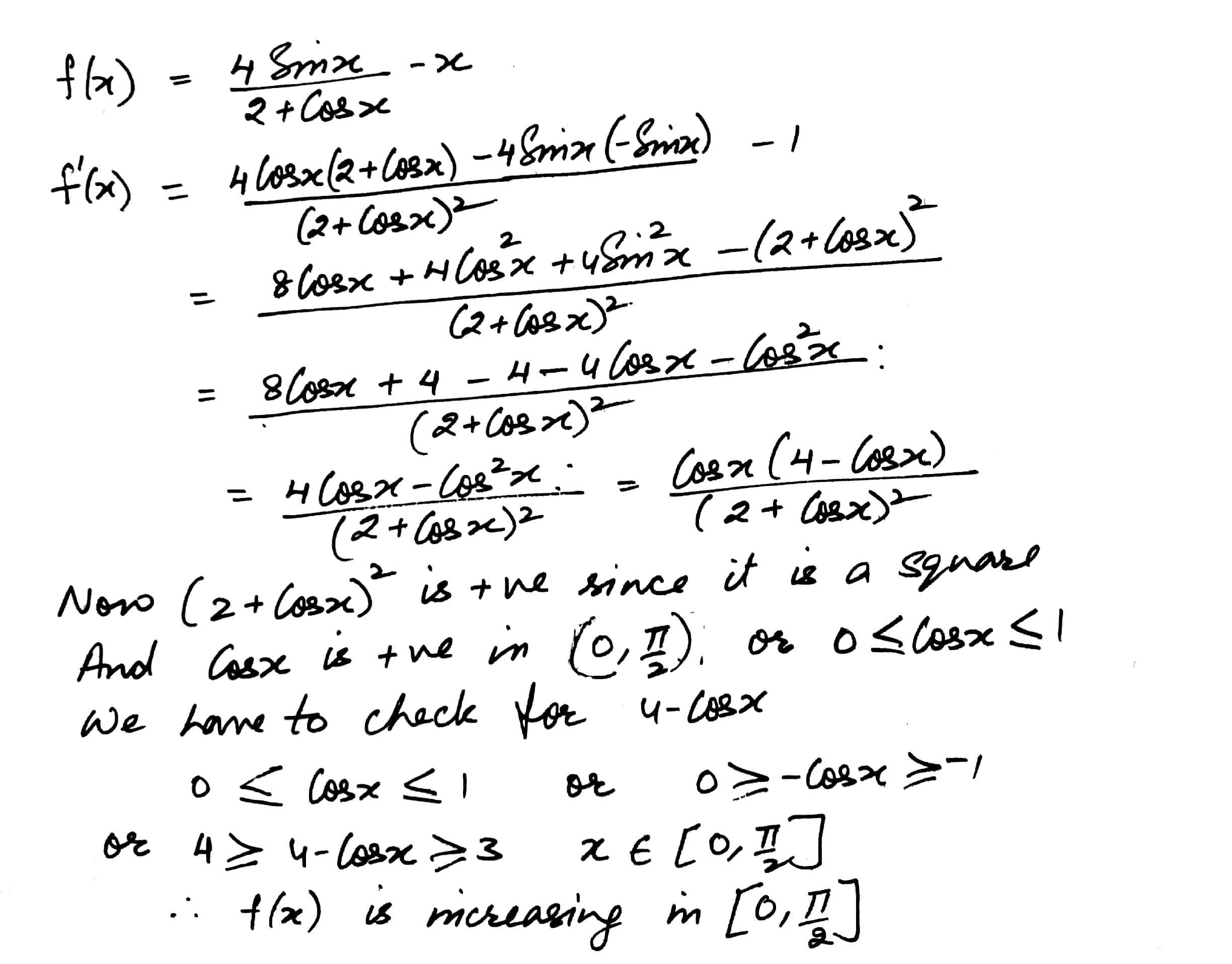5724

Mathematics Integration Level: Misc Level

Prove that the function f given by f(x) = Sin x is

(i)  strictly increasing on

(ii)  strictly decreasing on

(iii)  neither increasing nor decreasing in (0,)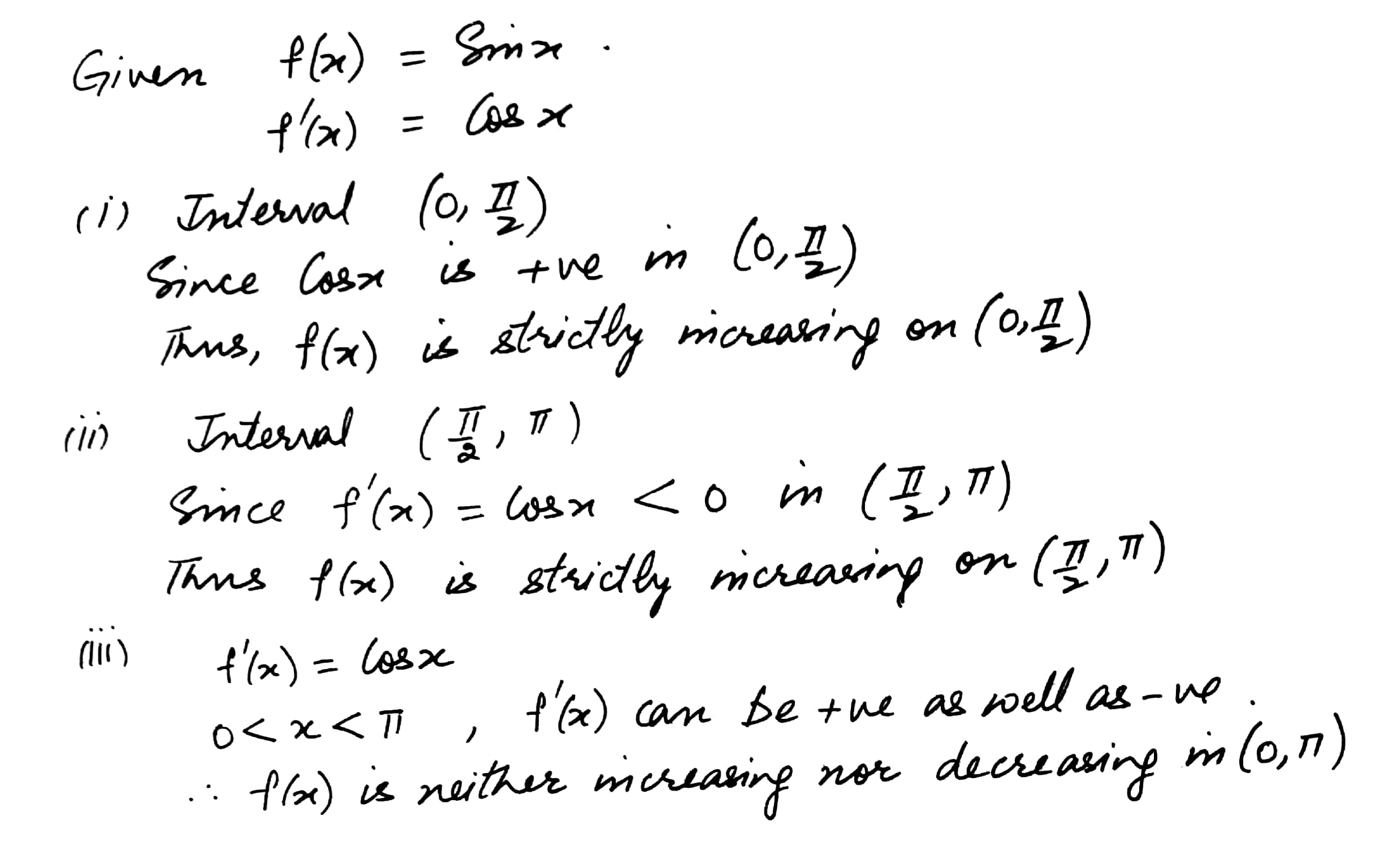5723

Mathematics Integration Level: Misc Level

Prove that the function f given by f(x) = log Cos x is strictly decreasing on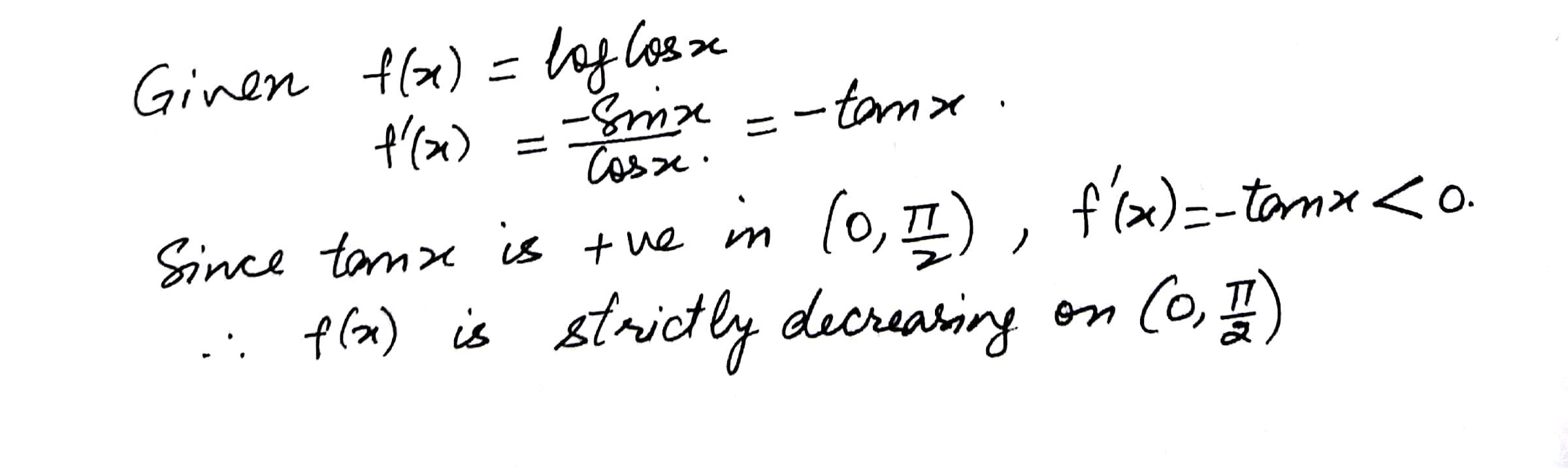5722

Mathematics Integration Level: Misc Level

Show that the function f given by f(x) = x3 - 3x2 + 4x, xR, is strictly increasing on R5721

Mathematics Integration Level: Misc Level

Show that the function f(x) = log x is strictly increasing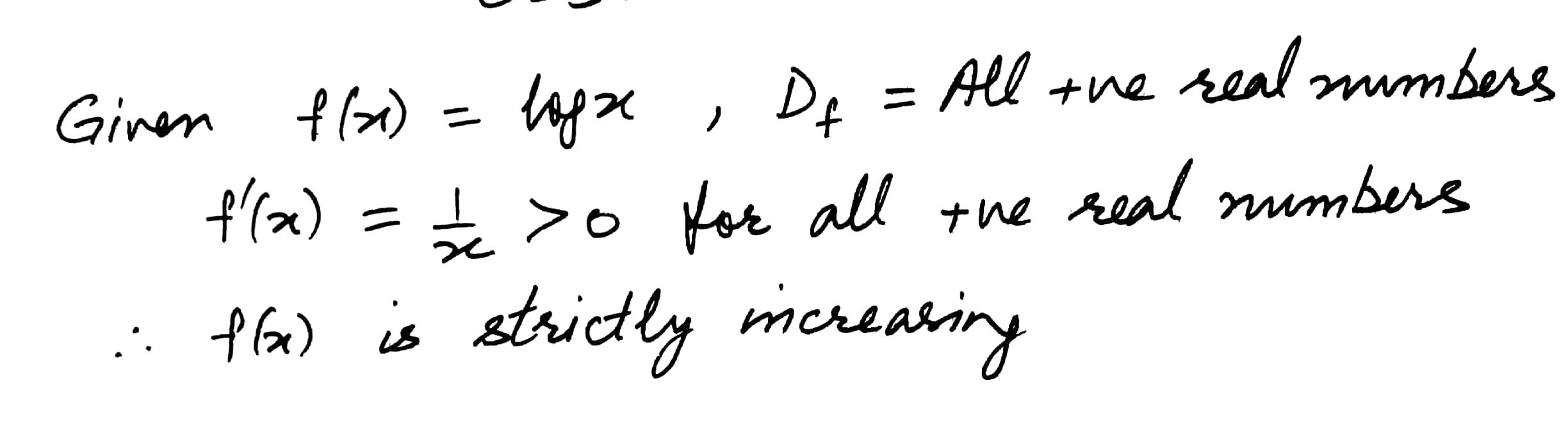5719

Mathematics Integration Level: Misc Level

Show that the function f(x) = 7x -3 is strictly increasing on R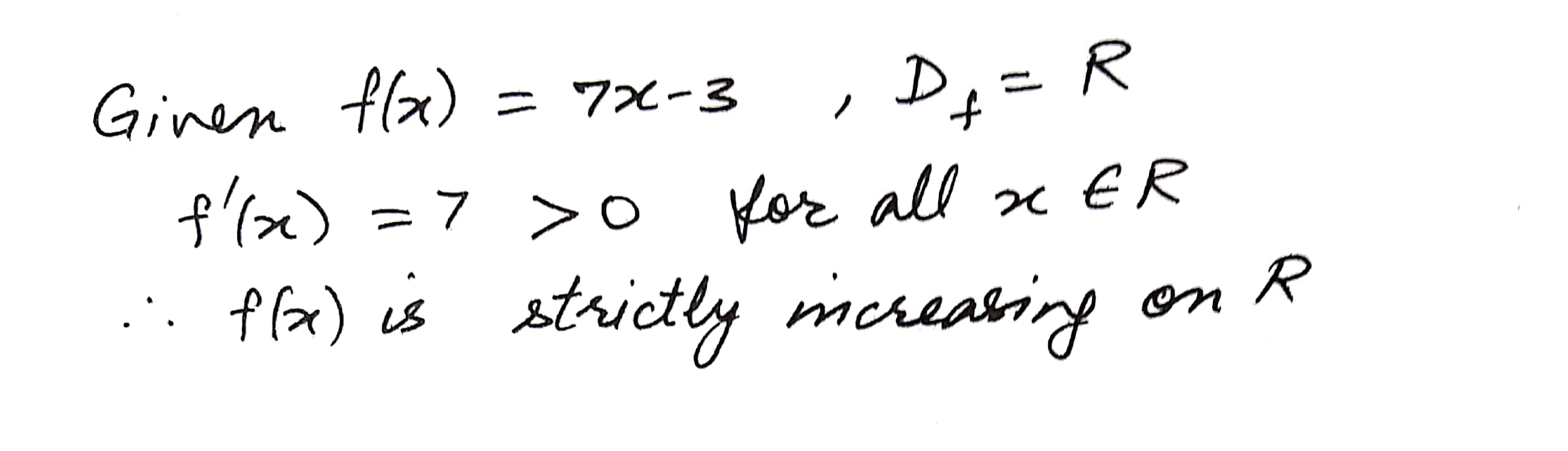Displaying 31-45 of 195 results.